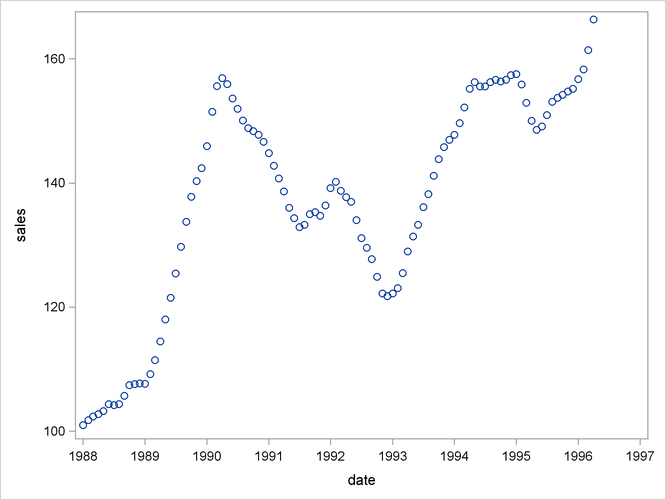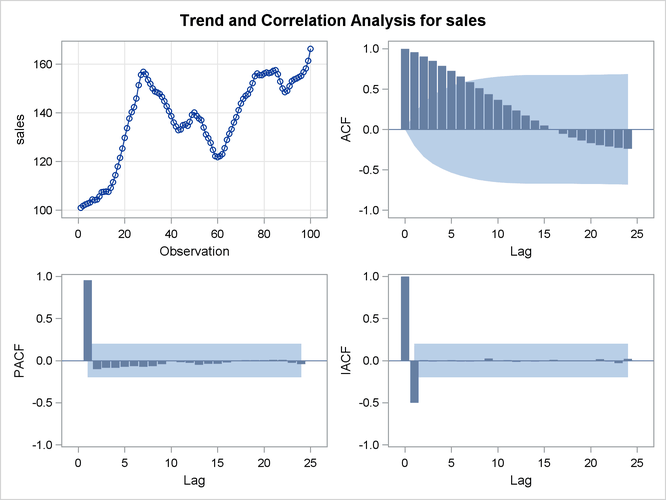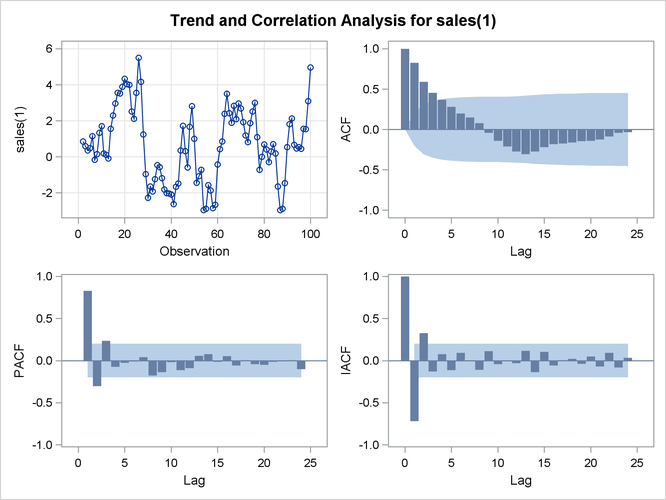### Identification Stage

Suppose you have a variable called `SALES` that you want to forecast. The following example illustrates ARIMA modeling and forecasting by using a simulated data set `TEST` that contains a time series `SALES` generated by an ARIMA(1,1,1) model. The output produced by this example is explained in the following sections. The simulated `SALES` series is shown in Figure 7.1.

```proc sgplot data=test;
scatter y=sales x=date;
run;
```

Figure 7.1: Simulated ARIMA(1,1,1) Series SALES#### Using the IDENTIFY Statement

You first specify the input data set in the PROC ARIMA statement. Then, you use an IDENTIFY statement to read in the `SALES` series and analyze its correlation properties. You do this by using the following statements:

```proc arima data=test ;
identify var=sales nlag=24;
run;
```
##### Descriptive Statistics

The IDENTIFY statement first prints descriptive statistics for the `SALES` series. This part of the IDENTIFY statement output is shown in Figure 7.2.

Figure 7.2: IDENTIFY Statement Descriptive Statistics Output

The ARIMA Procedure

Name of Variable = sales
Mean of Working Series 137.3662
Standard Deviation 17.36385
Number of Observations 100

##### Autocorrelation Function Plots

The IDENTIFY statement next produces a panel of plots used for its autocorrelation and trend analysis. The panel contains the following plots:

• the time series plot of the series

• the sample autocorrelation function plot (ACF)

• the sample inverse autocorrelation function plot (IACF)

• the sample partial autocorrelation function plot (PACF)

This correlation analysis panel is shown in Figure 7.3.

Figure 7.3: Correlation Analysis of SALESThese autocorrelation function plots show the degree of correlation with past values of the series as a function of the number of periods in the past (that is, the lag) at which the correlation is computed.

The NLAG= option controls the number of lags for which the autocorrelations are shown. By default, the autocorrelation functions are plotted to lag 24.

Most books on time series analysis explain how to interpret the autocorrelation and the partial autocorrelation plots. See the section The Inverse Autocorrelation Function for a discussion of the inverse autocorrelation plots.

By examining these plots, you can judge whether the series is stationary or nonstationary. In this case, a visual inspection of the autocorrelation function plot indicates that the `SALES` series is nonstationary, since the ACF decays very slowly. For more formal stationarity tests, use the STATIONARITY= option. (See the section Stationarity.)

##### White Noise Test

The last part of the default IDENTIFY statement output is the check for white noise. This is an approximate statistical test of the hypothesis that none of the autocorrelations of the series up to a given lag are significantly different from 0. If this is true for all lags, then there is no information in the series to model, and no ARIMA model is needed for the series.

The autocorrelations are checked in groups of six, and the number of lags checked depends on the NLAG= option. The check for white noise output is shown in Figure 7.4.

Figure 7.4: IDENTIFY Statement Check for White Noise

Autocorrelation Check for White Noise
To Lag Chi-Square DF Pr > ChiSq Autocorrelations
6 426.44 6 <.0001 0.957 0.907 0.852 0.791 0.726 0.659
12 547.82 12 <.0001 0.588 0.514 0.440 0.370 0.303 0.238
18 554.70 18 <.0001 0.174 0.112 0.052 -0.004 -0.054 -0.098
24 585.73 24 <.0001 -0.135 -0.167 -0.192 -0.211 -0.227 -0.240

In this case, the white noise hypothesis is rejected very strongly, which is expected since the series is nonstationary. The p-value for the test of the first six autocorrelations is printed as <0.0001, which means the p-value is less than 0.0001.

#### Identification of the Differenced Series

Since the series is nonstationary, the next step is to transform it to a stationary series by differencing. That is, instead of modeling the `SALES` series itself, you model the change in `SALES` from one period to the next. To difference the `SALES` series, use another IDENTIFY statement and specify that the first difference of `SALES` be analyzed, as shown in the following statements:

```proc arima data=test;
identify var=sales(1);
run;
```

The second IDENTIFY statement produces the same information as the first, but for the change in `SALES` from one period to the next rather than for the total `SALES` in each period. The summary statistics output from this IDENTIFY statement is shown in Figure 7.5. Note that the period of differencing is given as 1, and one observation was lost through the differencing operation.

Figure 7.5: IDENTIFY Statement Output for Differenced Series

The ARIMA Procedure

Name of Variable = sales
Period(s) of Differencing 1
Mean of Working Series 0.660589
Standard Deviation 2.011543
Number of Observations 99
Observation(s) eliminated by differencing 1

The autocorrelation plots for the differenced series are shown in Figure 7.6.

Figure 7.6: Correlation Analysis of the Change in SALESThe autocorrelations decrease rapidly in this plot, indicating that the change in `SALES` is a stationary time series.

The next step in the Box-Jenkins methodology is to examine the patterns in the autocorrelation plot to choose candidate ARMA models to the series. The partial and inverse autocorrelation function plots are also useful aids in identifying appropriate ARMA models for the series.

In the usual Box-Jenkins approach to ARIMA modeling, the sample autocorrelation function, inverse autocorrelation function, and partial autocorrelation function are compared with the theoretical correlation functions expected from different kinds of ARMA models. This matching of theoretical autocorrelation functions of different ARMA models to the sample autocorrelation functions computed from the response series is the heart of the identification stage of Box-Jenkins modeling. Most textbooks on time series analysis, such as Pankratz (1983), discuss the theoretical autocorrelation functions for different kinds of ARMA models.

Since the input data are only a limited sample of the series, the sample autocorrelation functions computed from the input series only approximate the true autocorrelation function of the process that generates the series. This means that the sample autocorrelation functions do not exactly match the theoretical autocorrelation functions for any ARMA model and can have a pattern similar to that of several different ARMA models. If the series is white noise (a purely random process), then there is no need to fit a model. The check for white noise, shown in Figure 7.7, indicates that the change in `SALES` is highly autocorrelated. Thus, an autocorrelation model, for example an AR(1) model, might be a good candidate model to fit to this process.

Figure 7.7: IDENTIFY Statement Check for White Noise

Autocorrelation Check for White Noise
To Lag Chi-Square DF Pr > ChiSq Autocorrelations
6 154.44 6 <.0001 0.828 0.591 0.454 0.369 0.281 0.198
12 173.66 12 <.0001 0.151 0.081 -0.039 -0.141 -0.210 -0.274
18 209.64 18 <.0001 -0.305 -0.271 -0.218 -0.183 -0.174 -0.161
24 218.04 24 <.0001 -0.144 -0.141 -0.125 -0.085 -0.040 -0.032